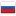Please subscribe to the official Codeforces channel in Telegram via the link https://t.me/codeforces_official. ×

Разбор задач Educational Codeforces Round 9

Revision en5, by Edvard, 2016-03-02 17:29:46

### 632A - Бабушка Лаура и яблоки

The problem was suggested by unprost.

Consider the process from the end. The last buyer will always buy a half of an apple and get a half for free (so the last string always is halfplus). After that each buyer increases the number of apples twice and also maybe by one. So we simply have the binary presentation of the number of apples from the end. To calculate the answer we should simply restore that value from the end and also calculate the total money grandma should have.

С++ solution by me.

Complexity: O(p).

### 632B - Алиса, Боб, две команды

The problem was suggested by Lewin Gan Lewin.

Let's calculate the prefix sums for all numbers (and store it in array s1) and for numbers with letter B (and store it in array s2). Now we can find the sum of all numbers in any segment in O(1) time and the sum of numbers with letter B.

Let's iterate over prefix or suffix to flip and calculate the sum in that case by formulas: sum(s1, 0, n - 1) + sum(s2, 0, i) - 2·sum(s1, 0, i) for prefixes and sum(s1, 0, n - 1) + sum(s2, i, n - 1) - 2·sum(s1, i, n - 1) for suffixes.

C++ solution by me.

Complexity: O(n).

### 632C - Наименьшая конкатенация строк

The problem was suggested by Lewin Gan Lewin. The proof of the transitivity also belongs to him.

Let's sort all the strings by comparator a + b < b + a and concatenate them. Let's prove that it's the optimal answer. Let that operator be transitive (so if). Consider an optimal answer with two strings in reverse order by that operator. Because of the transitivity of operator we can assume that pair of strings are neighbouring. But then we can swap them and get the better answer.

Let's prove the transitivity of operator. Consider the strings as the 26-base numbers. Then the relation a + b < b + a equivalent to. The last is simply the relation between real numbers. So we proved the transitivity of the relation a + b < b + a.

C++ solution by me.

Complexity: O(nLlogn), where L is the maximal string length.

### 632D - Самая длинная подпоследовательность

The problem was suggested by Denis Bezrukov pitfall.

Let cntx be the number of occurences of the number x in the given array (easy to see that we can ignore the numbers greater than m). Let's iterate overand 1 ≤ k, x·k ≤ m and increase the value in the position k·x in some array z by the value cntx. So the value zl equals the number of numbers in the given array which divide l. Let's find the minimal l with the maximum value zl (1 ≤ l ≤ m). Easy to see that the answer to the problem is the numbers which divide l.

Let's calculate the complexity of the solution. The number of the pairs (k, x) we can bound with the value.

C++ solution by me.

Complexity: O(n + mlogm).

### 632F - Волшебная матрица

The problem was suggested by Lewin Gan Lewin. The solution and proof also belongs to him.

Consider the undirected complete graph with n nodes, with an edge between nodes i, j with cost aij. Let Bij denote the minimum possible value of the max edge of a path from i to j. We know that aij ≥ Bij by definition.

If the matrix is magic, we can choose arbitrary k1, k2, ..., km such that aij ≤ max(ai, k1, ak1, k2, ..., akm, j) by repeating invocations of the inequality given. Also, you can show that if this inequality is satisfied, then the matrix is magic (by choosing an m = 1 and k1 arbitrary).

So, this shows that the matrix is magic if and only if aij ≤ Bij. Thus, combining with aij ≥ Bij, we have aij = Bij.

We need a fast way to compute Bij for all pairs i, j. This can be computed as the MST, as the path in the MST minimizes the max edge between all pairs of nodes. So, the algorithm works as follows. First, find the MST on the complete graph. Then, the matrix is magic if and only if the max edge on the path between i, j in the MST is exactly equal to ai, j. Also you shouldn't forget to check symmetry of the matrix and diagonal for zeros.

P.S.: Unfortunately we couldn't increase the value n in this problem: the tests already had the size about 67MB and they couldn't be given with generator. So most of the users who solved this problem uses bitset-s. The complexity of their solution is, where b = 32 or b = 64.

Complexity: O(n2logn) or O(n2).#### History

RevisionsRev. Lang. By When Δ Comment
en7Edvard 2016-03-03 02:14:37 138
ru12Edvard 2016-03-03 02:04:28 140
ru11Edvard 2016-03-02 17:52:11 122
en6Edvard 2016-03-02 17:51:16 2062
en5Edvard 2016-03-02 17:29:46 963
en4Edvard 2016-03-02 17:17:52 1105
ru10Edvard 2016-03-02 17:05:02 180
en3Edvard 2016-03-02 17:03:47 748
en2Edvard 2016-03-02 16:58:00 686
ru9Edvard 2016-03-02 03:33:59 39
en1Edvard 2016-03-02 03:33:00 1838 Initial revision for English translation
ru8Edvard 2016-03-02 03:24:21 2035 Мелкая правка: '_2},\ldots\n,a_{k_m,j}' -
ru7Edvard 2016-03-02 02:55:42 4
ru6Edvard 2016-03-02 02:46:07 2299
ru5Edvard 2016-03-02 02:11:36 2
ru4Edvard 2016-03-02 02:08:48 870 Мелкая правка: 'руков [used:pitfall].' -
ru3Edvard 2016-03-02 01:36:50 1379 Мелкая правка: 'ость: $O(n logn l)$, где $l$ --- наиб' -
ru2Edvard 2016-03-02 01:14:49 776
ru1Edvard 2016-03-02 01:03:34 748 Первая редакция (опубликовано)